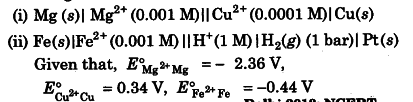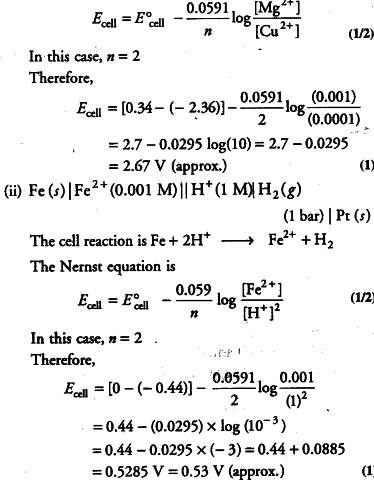# Write the Nernst equation and emf of the following cell at 298 K

Write the Nernst equation and emf of the following cell at 298 K.(i) For the given cell, the half-cell reactions will be given as below:

At anode Mg ------> {{Mg}^{2+}} + {{2e}^{-}}
At cathode {{Cu}^{2+}} + {{2e}^{-}} ------> Cu

Therefore, the overall cell reaction will be

Mg + {{Cu}^{2+}} -------> {{Mg}^{2+}} + Cu

The Nernst equation is